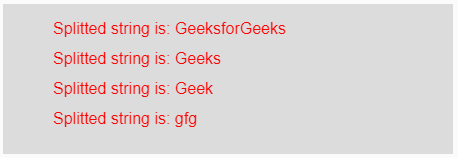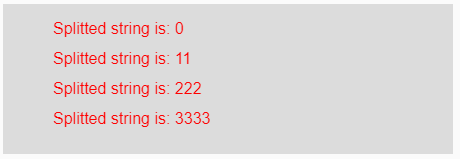# p5.js | split() function

The split() function in p5.js is used to break the input string into pieces using a delimiter. This delimiter could be any string or symbol used between each piece of the input string.

Syntax:

`split(String, Delimiter)`

Parameters: This function accepts two parameters which are described below:

• String: This is the input string which are to be splitted.
• Delimiter: This is any string or symbol used to separate the data of the input string.

Return Value: It returns the splitted data of the input string.

Below programs illustrate the split() function in p5.js.

Example 1: This example uses split() function to break the input string into pieces of substring using delemeter.

 `function` `setup() {  ` `  `  `    ``// Creating Canvas size ` `    ``createCanvas(450, 150);  ` `}  ` `function` `draw() {  ` `      `  `    ``// Set the background color  ` `    ``background(220);  ` `    `  `    ``// Initializing the Strings ` `    ``let String = ``'GeeksforGeeks/Geeks/Geek/gfg'``;   ` `     `  `    ``// Calling to split() function. ` `    ``let A = split(String, ``'/'``); ` `     `  `    ``// Set the size of text  ` `    ``textSize(16);  ` `      `  `    ``// Set the text color  ` `    ``fill(color(``'red'``));  ` `    `  `    ``// Getting splitted string ` `    ``text(``"Splitted string is: "` `+ A, 50, 30);   ` `    ``text(``"Splitted string is: "` `+ A, 50, 60);   ` `    ``text(``"Splitted string is: "` `+ A, 50, 90);   ` `    ``text(``"Splitted string is: "` `+ A, 50, 120); ` `}  `

Output:Example 2: This example uses split() function to break the input string into pieces of substring using delemeter.

 `function` `setup() {  ` `  `  `    ``// Creating Canvas size ` `    ``createCanvas(450, 150);  ` `}  ` `function` `draw() {  ` `      `  `    ``// Set the background color  ` `    ``background(220);  ` `    `  `    ``// Initializing the Strings ` `    ``let String = ``'0&11&222&3333'``;   ` `     `  `    ``// Calling to split() function. ` `    ``let A = split(String, ``'&'``); ` `     `  `    ``// Set the size of text  ` `    ``textSize(16);  ` `      `  `    ``// Set the text color  ` `    ``fill(color(``'red'``));  ` `    `  `    ``// Getting splitted string ` `    ``text(``"Splitted string is: "` `+ A, 50, 30);   ` `    ``text(``"Splitted string is: "` `+ A, 50, 60);   ` `    ``text(``"Splitted string is: "` `+ A, 50, 90);   ` `    ``text(``"Splitted string is: "` `+ A, 50, 120); ` `}  `

Output:Reference: https://p5js.org/reference/#/p5/split

My Personal Notes arrow_drop_upCheck out this Author's contributed articles.

If you like GeeksforGeeks and would like to contribute, you can also write an article using contribute.geeksforgeeks.org or mail your article to contribute@geeksforgeeks.org. See your article appearing on the GeeksforGeeks main page and help other Geeks.

Please Improve this article if you find anything incorrect by clicking on the "Improve Article" button below.

Article Tags :

Be the First to upvote.

Please write to us at contribute@geeksforgeeks.org to report any issue with the above content.